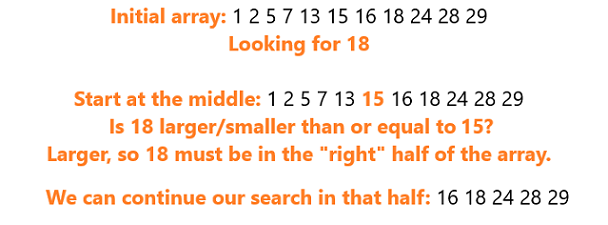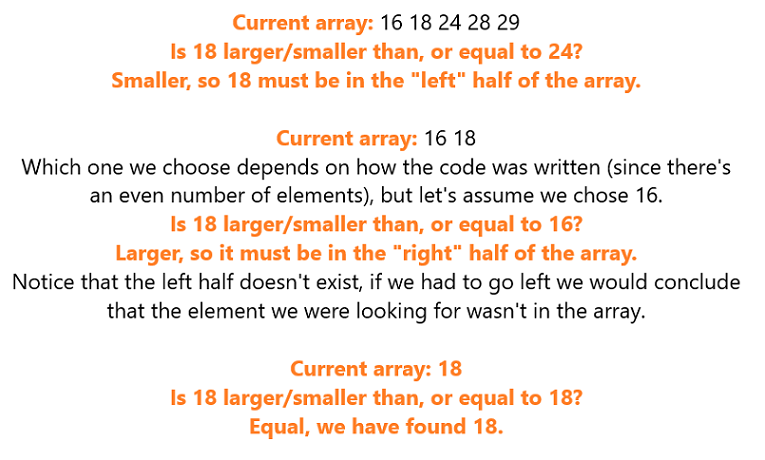Binary Search in Python - Stack Abuse

# Binary Search in Python### Introduction

In this article, we'll be diving into the idea behind and Python implementation of Binary Search.

Binary Search is an efficient search algorithm that works on sorted arrays. It's often used as one of the first examples of algorithms that run in logarithmic time (O(logn)) because of its intuitive behavior, and is a fundamental algorithm in Computer Science.

### Binary Search - Example

Binary Search works on a divide-and-conquer approach and relies on the fact that the array is sorted to eliminate half of possible candidates in each iteration. More specifically, it compares the middle element of the sorted array to the element it's searching for in order to decide where to continue the search.

If the target element is larger than the middle element - it can't be located in the first half of the collection so it's discarded. The same goes the other way around.

Note: If the array has an even number of elements, it doesn't matter which of the two "middle" elements we use to begin with.

Let's look at an example quickly before we continue to explain how binary search works:As we can see, we know for sure that, since the array is sorted, x is not in the first half of the original array.

When we know in which half of the original array x is, we can repeat this exact process with that half and split it into halves again, discarding the half that surely doesn't contain x:We repeat this process until we end up with a subarray that contains only one element. We check whether that element is x. If it is - we found x, if it isn't - x doesn't exist in the array at all.

If you take a closer look at this, you can notice that in the worst-case scenario (x not existing in the array), we need to check a much smaller number of elements than we'd need to in an unsorted array - which would require something more along the lines of Linear Search, which is insanely inefficient.

To be more precise, the number of elements we need to check in the worst case is log2N where N is the number of elements in the array.

This makes a bigger impact the bigger the array is:

If our array had 10 elements, we would need to check only 3 elements to either find x or conclude it's not there. That's 33.3%.

However, if our array had 10,000,000 elements we would only need to check 24 elements. That's 0.0002%.

### Binary Search Implementation

Binary Search is a naturally recursive algorithm, since the same process is repeated on smaller and smaller arrays until an array of size 1 has been found. However, there is of course an iterative implementation as well, and we will be showing both approaches.

#### Recursive

Let's start off with the recursive implementation as it's more natural:

def binary_search_recursive(array, element, start, end):
if start > end:
return -1

mid = (start + end) // 2
if element == array[mid]:
return mid

if element < array[mid]:
return binary_search_recursive(array, element, start, mid-1)
else:
return binary_search_recursive(array, element, mid+1, end)


Let's take a closer look at this code. We exit the recursion if the start element is higher than the end element:

if start > end:
return -1


This is because this situation occurs only when the element doesn't exist in the array. What happens is that we end up with only one element in the current sub-array, and that element doesn't match the one we're looking for.

At this point, start is equal to end. However, since element isn't equal to array[mid], we "split" the array again in such a way that we either decrease end by 1, or increase start by one, and the recursion exists on that condition.

We could have done this using a different approach:

if len(array) == 1:
if element == array[mid]:
return mid
else:
return -1


The rest of the code does the "check middle element, continue search in the appropriate half of the array" logic. We find the index of the middle element and check whether the element we're searching for matches it:

mid = (start + end) // 2
if elem == array[mid]:
return mid


## Better understand your data with visualizations

•  30-day no-questions refunds
•  Beginner to Advanced
•  Updated regularly (update June 2021)
•  New bonus resources and guides

If it doesn't, we check whether the element is smaller or larger than the middle element:

if element < array[mid]:
# Continue the search in the left half
return binary_search_recursive(array, element, start, mid-1)
else:
# Continue the search in the right half
return binary_search_recursive(array, element, mid+1, end)


Let's go ahead and run this algorithm, with a slight modification so that it prints out which subarray it's working on currently:

element = 18
array = [1, 2, 5, 7, 13, 15, 16, 18, 24, 28, 29]

print("Searching for {}".format(element))
print("Index of {}: {}".format(element, binary_search_recursive(array, element, 0, len(array))))


Running this code will result in:

Searching for 18
Subarray in step 0:[1, 2, 5, 7, 13, 15, 16, 18, 24, 28, 29]
Subarray in step 1:[16, 18, 24, 28, 29]
Subarray in step 2:[16, 18]
Subarray in step 3:
Index of 18: 7


It's clear to see how it halves the search space in each iteration, getting closer and closer to the element we're looking for. If we tried searching for an element that doesn't exist in the array, the output would be:

Searching for 20
Subarray in step 0: [4, 14, 16, 17, 19, 21, 24, 28, 30, 35, 36, 38, 39, 40, 41, 43]
Subarray in step 1: [4, 14, 16, 17, 19, 21, 24, 28]
Subarray in step 2: [19, 21, 24, 28]
Subarray in step 3: 
Index of 20: -1


And just for the fun of it, we can try searching some large arrays and seeing how many steps it takes Binary Search to figure out whether a number exists:

Searching for 421, in an array with 200 elements
Search finished in 6 steps. Index of 421: 169

Searching for 1800, in an array with 1500 elements
Search finished in 11 steps. Index of 1800: -1

Searching for 3101, in an array with 3000 elements
Search finished in 8 steps. Index of 3101: 1551


#### Iterative

The iterative approach is very simple and similar to the recursive approach. Here, we just perform the checks in a while loop:

def binary_search_iterative(array, element):
mid = 0
start = 0
end = len(array)
step = 0

while (start <= end):
print("Subarray in step {}: {}".format(step, str(array[start:end+1])))
step = step+1
mid = (start + end) // 2

if element == array[mid]:
return mid

if element < array[mid]:
end = mid - 1
else:
start = mid + 1
return -1


Let's populate an array and search for an element within it:

array = [1, 2, 5, 7, 13, 15, 16, 18, 24, 28, 29]

print("Searching for {} in {}".format(element, array))
print("Index of {}: {}".format(element, binary_search_iterative(array, element)))


Running this code gives us the output of:

Searching for 18 in [1, 2, 5, 7, 13, 15, 16, 18, 24, 28, 29]
Subarray in step 0: [1, 2, 5, 7, 13, 15, 16, 18, 24, 28, 29]
Subarray in step 1: [16, 18, 24, 28, 29]
Subarray in step 2: [16, 18]
Subarray in step 3: 
Index of 18: 7


### Conclusion

Binary Search is an incredible algorithm to use on large, sorted arrays, or whenever we plan to search for elements repeatedly in a single array.

The cost of sorting the array once and then using Binary Search to find elements in it multiple times is far better than using Linear Search on an unsorted array just so we could avoid the cost of sorting it.

If we're sorting the array and searching for an element just once, it's more efficient to just do a Linear Search on the unsorted array.

If you'd like to read about Sorting Algorithms in Python, we've got you covered!

Last Updated: June 3rd, 2020

## Improve your dev skills!

Get tutorials, guides, and dev jobs in your inbox.

No spam ever. Unsubscribe at any time. Read our Privacy Policy.

Olivera PopovićAuthor

LinkedIn: https://rs.linkedin.com/in/227503161
If you need any help - post it in the comments :) That way someone else can reply if I'm busy.

# Prepping for an interview?

• Improve your skills by solving one coding problem every day
• Get the solutions the next morning via email
• Practice on actual problems asked by top companies, like:

# Better understand your data with visualizations

•  30-day no-questions refunds
•  Beginner to Advanced
•  Updated regularly (update June 2021)
•  New bonus resources and guides

© 2013-2021 Stack Abuse. All rights reserved.